moveit2 The MoveIt Motion Planning Framework for ROS 2.
trajectory_processing::Trajectory Class Reference

`#include <time_optimal_trajectory_generation.h>`

## Public Member Functions

Trajectory (const Path &path, const Eigen::VectorXd &max_velocity, const Eigen::VectorXd &max_acceleration, double time_step=0.001)
Generates a time-optimal trajectory. More...

~Trajectory ()

bool isValid () const
Call this method after constructing the object to make sure the trajectory generation succeeded without errors. If this method returns false, all other methods have undefined behavior. More...

double getDuration () const
Returns the optimal duration of the trajectory. More...

Eigen::VectorXd getPosition (double time) const
Return the position/configuration vector for a given point in time. More...

Eigen::VectorXd getVelocity (double time) const
Return the velocity vector for a given point in time. More...

Eigen::VectorXd getAcceleration (double time) const
Return the acceleration vector for a given point in time. More...

## Detailed Description

Definition at line 108 of file time_optimal_trajectory_generation.h.

## ◆ Trajectory()

 trajectory_processing::Trajectory::Trajectory ( const Path & path, const Eigen::VectorXd & max_velocity, const Eigen::VectorXd & max_acceleration, double time_step = `0.001` )

Generates a time-optimal trajectory.

Definition at line 318 of file time_optimal_trajectory_generation.cpp.

## ◆ ~Trajectory()

 trajectory_processing::Trajectory::~Trajectory ( )

Definition at line 370 of file time_optimal_trajectory_generation.cpp.

## ◆ getAcceleration()

 Eigen::VectorXd trajectory_processing::Trajectory::getAcceleration ( double time ) const

Return the acceleration vector for a given point in time.

Definition at line 857 of file time_optimal_trajectory_generation.cpp.

Here is the call graph for this function: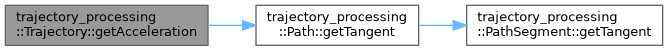Here is the caller graph for this function: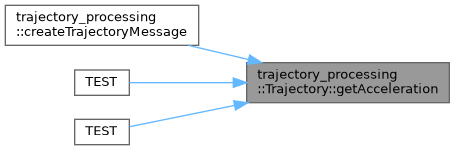## ◆ getDuration()

 double trajectory_processing::Trajectory::getDuration ( ) const

Returns the optimal duration of the trajectory.

Definition at line 795 of file time_optimal_trajectory_generation.cpp.

Here is the caller graph for this function: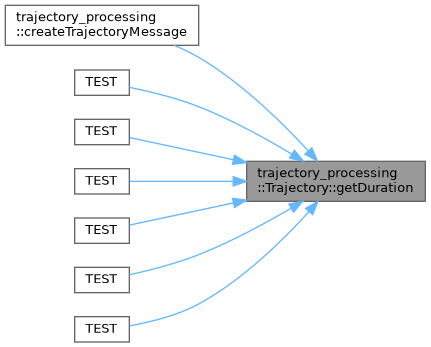## ◆ getPosition()

 Eigen::VectorXd trajectory_processing::Trajectory::getPosition ( double time ) const

Return the position/configuration vector for a given point in time.

Definition at line 823 of file time_optimal_trajectory_generation.cpp.

Here is the call graph for this function: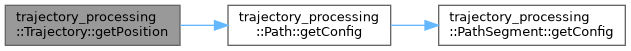Here is the caller graph for this function: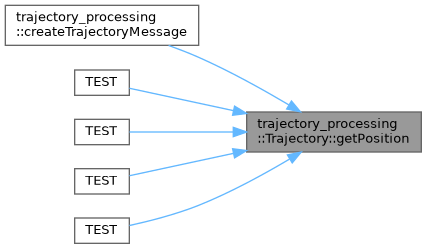## ◆ getVelocity()

 Eigen::VectorXd trajectory_processing::Trajectory::getVelocity ( double time ) const

Return the velocity vector for a given point in time.

Definition at line 840 of file time_optimal_trajectory_generation.cpp.

Here is the call graph for this function: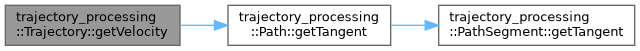Here is the caller graph for this function: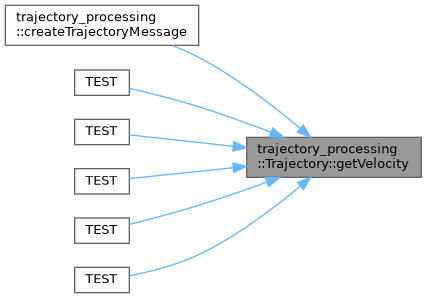## ◆ isValid()

 bool trajectory_processing::Trajectory::isValid ( ) const

Call this method after constructing the object to make sure the trajectory generation succeeded without errors. If this method returns false, all other methods have undefined behavior.

Definition at line 790 of file time_optimal_trajectory_generation.cpp.

Here is the caller graph for this function:The documentation for this class was generated from the following files: